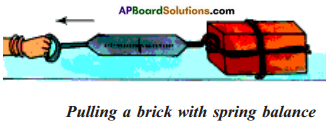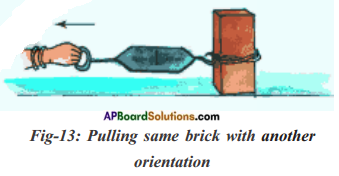AP State Syllabus AP Board 8th Class Physical Science Important Questions Chapter 2 Friction

## AP State Syllabus 8th Class Physical Science Important Questions 2nd Lesson Friction

### 8th Class Physical Science 2nd Lesson Friction 1 Mark Important Questions and Answers

Question 1.
What is frictional force?
The force which opposes the relative motion of two surfaces of bodies which are in contact is called frictional force.

Question 2.
What is static friction?
Static friction is the friction which comes into play when surfaces of objects are at rest relative to each otherQuestion 3.
What is sliding friction?
Sliding friction is the friction which comes into play when the surface of one object moves relative to the surface of another object.

Question 4.
What is rolling friction?
When one body rolls over the surface of the other body the friction offered is called rolling friction.

Question 5.
Write the order of friction.
Static friction > Sliding friction > Rolling friction.

Question 6.
What are lubricants?
The substances which reduce friction are called lubricants.

Question 7.
What are the factors which influence friction?
The factors which influence friction are: 1) Nature of surface, 2) Normal force.

Question 8.
What is drag?
The frictional force exerted by fluids is called drag.

Question 9.
What are the factors which frictional force of an object in fluid depends on?
The frictional force of an object in a fluid depepds on its speed with respect to fluid and on the shape of the object and nature of the fluid.

Question 10.
If friction was absent can you light a match stick?
No, to light a match stick it is to be rubbed against the rough surface of a match box.Question 11.
Hard metal balls are used between rolling parts in a machine. How does it reduces friction?
Firstly it reduces the area of contact and reduces the friction. Secondly the hard balls experiences small forces of deformation that also reduce friction.

Question 12.
When a car is speeding on a level track, it enters on a concrete road from a tar road. What happens to its speed when the acceleration is unchanged?
The friction between the tyres and the concrete road is less than that on the tar road. Therefore, the car accelerates more on the concrete road.

Question 13.
A car moving on a concrete road entered on a glacier. The driver applied brakes. Describe its motion.
Because the friction is almost zero on the glacier, the car would not stop even though the tyres stop moving. That is the car skid forward.

Question 14.
Why do we use grease between moving parts of motor vehicles?
To reduce friction and increase the efficiency of motor vehicles.

Question 15.
What is effect of roughness on friction?
As the roughness of surface increases frictional force increases.

Question 16.
Why do Gymnasts apply some coarse substance on their hands?
Gymnasts apply some coarse substance on their hands to increase friction for better grip.

Question 17.
What is the effect of area of contact on frictional force?
Frictional force is independent of area of contact.Question 18.
Why do we need to change the tyres when its threading is worn out?
If we does not change the tyre then the frictional force decreases due to threading worn out tyre there by the vehicle may skid.

Question 19.
Why birds and fishes have special shapes?
Birds and fishes have to move about in fluids all the time so they have special shapes which would make them not to lose much energy in overcoming friction.

Question 20.
Why shape of aeroplane is similar to bird?
The shape of birds are useful in reducing the fluid friction so aeroplane shape was made similar to bird in order to reduce fluid friction.

Question 21.
How does life history of Leonardo Davinci inspire students?
Leonardo Davinci was concerned with laws of science and nature which greatly informed his work as a painter, sculptor, inventor and draft men. His versatile personality inspire students.

### 8th Class Physical Science 2nd Lesson Friction 2 Marks Important Questions and Answers

Question 1.
What is practical application of static friction?

1. The objects unable to move on their own is due to static friction otherwise no object be stationary at a particular place.
2. All the objects we used in our daily life are stationary because they unable to over-come static friction.

Question 2.
Why frictional force increases with roughness?

1. Friction caused by the irregularities on the two surfaces which are in contact.
2. Irregularities on the two surfaces lock into one another and we have to apply force to overcome interlocking to move any surface.
3. On roughness surfaces there exists a large number of irregularities so the force of friction is greater if a rough surface is involved.Question 3.
Give few examples for Sliding friction.

1. The trolley moves on the floor.
2. The marbles or any spherical objects slide on the ground or floor when thrown.
3. The tyres make sliding movement on roads which moves the vehicles.

Question 4.
What happens if we do not reduce friction in machines?
If we do not reduce friction in machines, then friction causes overheating and wearing out of moving parts of machinery.

Question 5.
What purposes are surved by using bearings in machines? Explain with daily life examples.
Ball bearings are used to reduce friction between rotating shafts of machine tools.

1. Ball bearings are used in fAnswer:
2. Ball bearings are also used in machines.

Question 6.
Give examples of practical application of static friction.

1. The static friction does not make the bodies move easily. So, the bodies are at rest.
2. The static friction helps the vehicles to come to rest.
3. The static friction helps us to escape from slippery.

Question 7.
Why does friction between two surfaces increase when they are pressed harder?
Since the friction is due to the interlocking of irregularities in the two surfaces which slide with respect to each other, it is obvious that the force of friction will increase if the two surfaces are pressed harder.

Question 8.
Describe some examples where heat is produced due to friction.

1. When we rub our hands together vigorously for few minutes we feel heat due to friction.
2. When we strike a matchstick, against rough surface, it catches fire due to friction.Question 9.
Why are the soles of our shoes and tyres of cars, trucks, etc. grooved?

1. It is done to provide the shoes better grip on the floor, so that one can move safely.
2. Similarly, the threaded tyres of cars, trucks and buldozers provide better grip with the ground.

Question 10.
Why heavier box apply more frictional force than lighter box?

1. We know that the force of friction is increased if the two surfaces are pressed harder.
2. A heavier box presses the ground harder to heavier box apply more frictional force.

Question 11.
Why it is easy to move an object in motion when compared with object at rest?

1. When the box start moving, the contact points on its surface, do not get enough time to lock into the contact points of floor.
2. So it is easy to move the box which already started to move.

Question 12.
What is the function of ridges on your palm and bottom of your feet?
Ridges of our palm help us to make firm grip whenever we hold by our hands – Ridges of the bottom of our feet help us to walk freely and we do not slip because of these.

Question 13.
On what factors does the fluid friction depend?

1. The frictional force on an object in a fluid depends on its speed with respect to the fluid.
2. The frictional force also depends on the shape of the object and the nature of the fluid.

Question 14.
Describe some examples where we have to increase friction.

1. We will increase friction by using brake pads in the brake system of bicycles and automobiles.
2. Kabaddi players rub their hands with the soil for an easy grip of their opponents.
3. Gymnasts apply some coarse substances on their hands to increase friction for better grip.Question 15.
Why is it easy to drag a mat over the floor when no weight is kept over it?

1. When weight is kept over the mat, more force will be applied.
2. Due to more force, interlocking of two surfaces will increase, so friction also increases.
3. That is why it is easy to drag a mat over the floor when no weight is kept over it.

Question 16.
Explain why we use ball bearings between the hub and axle of ceiling fan and bicycle?

1. Since the rolling friction is smaller than the sliding friction.
2. So sliding is replaced in most machines by rolling with the use of ball bearings.
3. Common examples are the use of ball bearings between the hub and axle of a ceiling fan and the bicycle.

Question 17.
Explain why Gymnasts apply some coarse substance on their hands?

1. Gymnasts apply some coarse substance on their hands to increase friction for better grip.
2. This is due to increasing the large number of irregularities (up & downs) in the contact area.

### 8th Class Physical Science 2nd Lesson Friction 4 Marks Important Questions and Answers

Question 1.
What are the forces acting on a moving body on a floor?
Along vertical direction: Weight of the body (W) or gravitational force acting vertically down.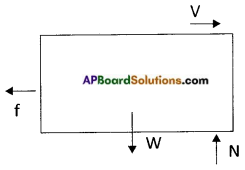Normal force (N) or reaction force applied by the floor vertically up.
As there is no change in the motion of the body f along vertical direction the net force along vertical direction is 0.
That is W – N = 0 ⇒ W = N
Along horizontal direction : In horizontal direction the speed of the body changing gradually due to force, applied by the floor on the body. The force applied by the floor on the body is called frictional force.Question 2.
What kinds of friction do you know and explain with suitable example.
Friction is of four kinds. They are:

1. Static friction: Friction which comes into play when surfaces of the objects are at rest relative to each other.
Ex: 1) We are applying force on a big rock but unable to move the rock due to its static friction.
2) Block at rest relative to the surface of trolley upto a certain limiting acceleration.
2. Sliding friction : The friction which comes into play when the surface of one object moves relative to the surface of another object.
Ex: When the applied force is more than limit of static friction, then the object starts to move which is example for sliding friction.
3. Rolling friction : When one body rolls over the surface of another body, the friction offered is called rolling friction.
Ex: It is easy to pull a suitcase which has rollers.
4. Fluid friction : Friction exerted by fluids like liquids and gases is called fluid friction. Ex : The shape of birds are such that they overcome the fluid friction.

Question 3.
A book placed on the surface of a table. The book is pushed in one direction. Draw the forces acting on the book and explain.
The weight acts downwards and normal force acts upward direction they balance each other.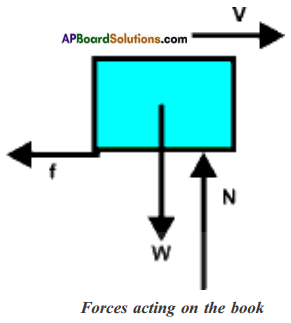Whereas frictional force acting opposite to the applied force.

Question 4.
Explain with one example that Frictional force is proportional to the Normal force.
Keep a brick on the horizontal floor tied to the spring balance. Pull the spring balance and note the frictional force.
Do the same experiment by adding one more brick above the first one.
Tie a brick to the spring balance and pull it to find the frictional force. The normal force acts on it provides a frictional force.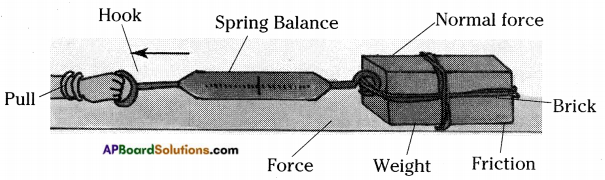When we pull a brick over another brick the normal force acting on them increases so that the frictional force increases.
∴ Friction °c Normal forceQuestion 5.
Scientists designed the shape of aeroplane using the technique of reducing fluid friction by observing birds shape. How would you appreciate?

1. Objects move through fluids, they have to overcome friction acting on them.
2. Birds and fishes have to move about in fluids all the time.
3. Their bodies must have evolved to shapes which would make them not to lose much energy in overcoming friction.
4. So aeroplanes designed in a special shape to overcome friction without loosing much energy.
Hence I appreciate the idea of scientists to overcome fluid friction by designing shape of aeroplane.

Question 6.
Conduct an experiment to findout the effect of roughness on frictional force.
Effect of roughness on frictional force:

1. Set up an inclined plane on the horizontal floor. Use a wooden board as inclined plane.
2. Put a mark at any point A on the inclined plane. Now let a pencil cell or ball move down from this point.
3. Note the distance covered by the pencil cell from the bottom of the inclined plane to point where it comes to stop.
4. Now, spread a cloth over the floor make sure that there are no wrinkles in the cloth.
5. Try again with pencil cell. Now note down the distance.
6. Distance covered by pencil cell is less in this case.
7. Thus we conclude that smoothness/ roughness of the surfaces of both the floor and the pencil cell could affect the distance travelled by it.

Question 7.
Collect information about the substances using to reduce the friction in different machines and prepare a report on it.

1. Adding lubricants to machinery parts.
2. Using roller type ball bearings.
3. Using air pressure to separate moving parts.
4. Using magnetic repulsion to separate moving parts.
5. Using materials that tend to slide easily over each other.
6. Regulate the temperature of the machine.
7. The use of drive shafts.Question 8.
Write an experiment to identify forces acting on a body and effect of frictional force.
Gently push a book or any object on a horizontal floor. It moves for a certain distance and stops due to the friction.
The friction acts in the opposite direction of the force exerted.
Now take the same object and do the activity on different surfaces smooth and rough.
The object moves a long distance on the smooth surface whereas it moves a little distance on the rough surface.
We come across that the friction is more on the rough surface and less on the smooth surface due to the reduction in the sliding friction.

Question 9.
Friction – an evil master and good servant to man. Describe.
Friction is the obstruction force acting in the opposite direction of the exerted force. It brings the following bad or evil impacts on the bodies.

1. Over heating and wearing out of moving parts in all machinery
2. Loss of efforts

In this way it is a bad or evil master to man.
But, without friction the moving of vehicles on the roads, walking, running, sitting, standing, etc. of us are not possible.
We are able to write with pen or pencil on the papers or walls or any surface is only due to the influence of friction. .
In this way it is a good master.

Question 10.
Does the friction is affected by smoothness of surface? Explain.

1. Friction is caused by the irregularities on the two surfaces in contact.
2. Even those surface which appear very smooth have a large number of minute irregularities on them.
3.  Irregularities on the two surfaces lock into another.
4. When we attempt to move any one surface over the other, we have to apply a force to overcome interlocking.
5. On rough surfaces, there are a larger number of irregularities.
6. So the force of friction is greater if a rough surface is involved.Question 11.
What would happen if there were no force of friction? Imagine and describe in your own words.

1. If there were no friction, many problems could have been arisen, as one will not able to walk if they were no friction at all.
2. One could not write with pen or pencil.
3. One could not write on blackboard with a chalk.
4. If any object just started moving, it would never stop.
5. Had there been no friction between the tyres of the automobiles and the road, they could not be started or stopped or turned to change the direction of motion.
6. One could not fix the nail in the wall or tie a knot.
7. Without friction no buildings could be constructed.

### 8th Class Physical Science 2nd Lesson Friction Important Questions and Answers

Question 1.
“Friction produce heat” – suggest an activity to prove this.

1. Rub your palms against each other for a few minutes.
2. You can feel heat in your palms.
3. Hence we can say that friction produces heat.

Question 2.
Write any one precaution which was taken by you while doing the experiment to understand the nature of friction.
Keep the weights to the hanger slowly and one by one.

Question 3.
Give any two examples of ways of reducing friction.
We can reduce friction,

1. by using lubricants in between frictional surfaces,
2. by using ball bearings in the rotating parts.

Question 4.
If there is no frictional force, assume the consequences in your classroom. Write any four of them.
If there is no friction force,

1. We cannot hold a pen to write on a book.
2. We cannot walk in the classroom.
3. Teacher cannot write with chalk-piece on the blackboard.
4. Heat does not produce when rub the palms against each other.

Question 2.
What is fluid friction? Explain why objects moving in fluids must have special shapes.

1. When bodies move through fluids, fluids exert a frictional force called drag or fluid friction.
2. To minimise fluid friction, objects moving in fluids must have special shapes.
3. For example, Birds and Fishes have to move about in fluids all the time, so they have special shapes which would make them not to lose much energy in over coming friction.
4. Another example is the shape of aeroplane was made similar to bird in order to reduce fluid friction.Question 3.
Imagine the friction has disappeared from the earth. What will happen? Explain.

1. If there were no friction, many problems could have been arisen, as one will not able to walk if they were no friction at all.
2. One could not write with pen or pencil.
3. One could not write on blackboard with a chalk.
4. If any object just started moving, it would never stop.
5. Had there been no friction between the tyres of the automobiles and the road, they could not be started or stopped or turned to change the direction of motion.
6. One could not fix the nail in the wall or tie a knot.
7. Without friction no buildings could be constructed.

Question 4.
Name any two types of frictions. Write one example for each.
(OR)
What kinds of friction do you know? Write the conditions needed for static friction between two objects and what way do you suggest to reduce friction?
Friction is of four kinds. They are

1. Static friction: Friction which comes into play when surfaces of the objects are at rest relative to each other.
Ex : 1) We are applying force on a big rock but unable to move the rock due to its static friction.
2) Block at rest relative to the surface of trolley upto a certain limiting acceleration.
2. Sliding friction: The friction which comes into play when the surface of one object moves relative to the surface of another object.
Ex : When the applied force is more than limit of static friction, then the object starts to move which is example for sliding friction.
3. Rolling friction: When one body rolls over the surface of another body, the friction offered is called rolling friction.
Ex : It is easy to pull a suitcase which has rollers.
4. Fluid friction: Friction exerted by fluids like liquids and gases is called fluid friction. Ex : The shape of birds are such that they over come the fluid friction.

Static friction is a self adjusting force. The following conditions are needed for static friction ;

1. Roughness of the surface of contact and
2. Normal force.
3. We can reduce the friction by applying oil or grease between the moving parts of a machine.
4. If we polish the rough surfaces, they become smooth and friction is reduced.
5. We can apply soap solution to the rough surfaces.
6. We can apply powder of small quantity on the surface.
7. By streamlining the bodies of the vehicles we can reduce air friction.Question 5.
Friction does not depends upon area of contact. How can you perform an activity to prove it?